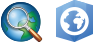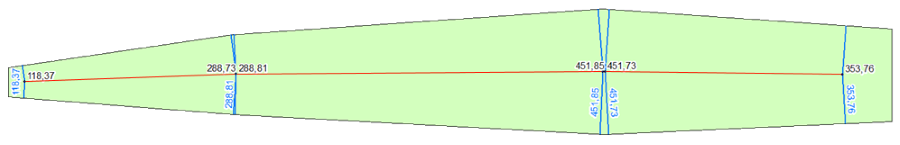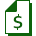# Calculate Polygon WidthCalculates polygon width values along polygon center lines.Available in XTools Pro for ArcMap and for ArcGIS Pro

The Calculate Polygon Width tool is provided to calculate width of polygon features. The output is the point feature class where each point is the node of the polygon center line. The calculated polygon width at the specified point is written to the attribute table of the output point feature class.When calculating the polygon width, the tool first calculates center line of the polygon, then extracts nodes from this line, after that calculates distances to the polygon border, and then writes the result to the point feature class. Since center lines of complex polygons may consist of several parts, the ID and the part number of center lines are written to the attribute table of the output feature class in addition to polygon width values.In terms of this tool, the polygon width is the sum of shortest distances from the center line nodes to the polygon borders.Paid tool. Try this and all other XTools Pro features free for 14 days or buy XTools Pro license now.